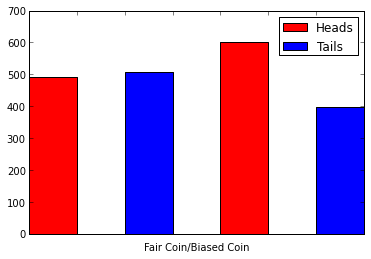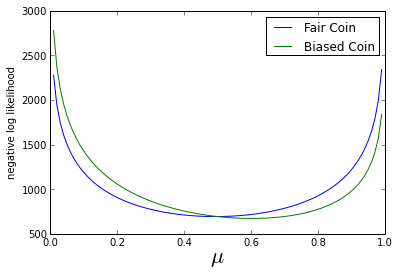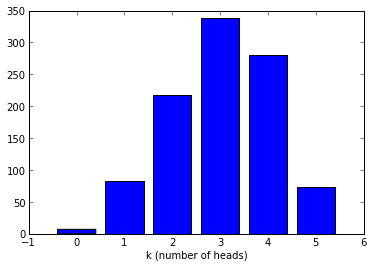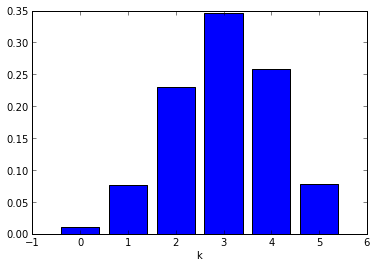In :
%pylab inline
from IPython.display import display, Math, Latex
maths = lambda s: display(Math(s))
latex = lambda s: display(Latex(s))

Welcome to pylab, a matplotlib-based Python environment [backend: module://IPython.zmq.pylab.backend_inline].

In :
class Coin(object):
"""
A simple coin class, that we can toss to get heads or tails,
it could be represented by a binary random variable x,
where x=1 (heads) or x=0 (tails)

Can have a biased coin, by changing mu, the probability x=1 (heads)
"""
def __init__(self, mu=0.5):
self.mu = mu

def toss(self, n=1):
return int_(random_sample(size=n) < self.mu)

def pmf(self, x):
# p(x=0|mu) = (1-mu)
# p(x=1|mu) = mu
# ie, the Bernoulli distribution
# which can be written as:
return self.mu**x * (1-self.mu)**(1-x)


In :
# Toss a fair coin and a biased coin 1000 times
faircoin = Coin(.5)
biasedcoin = Coin(.6)

nsamples = 1000

ftosses = faircoin.toss(nsamples)
btosses = biasedcoin.toss(nsamples)

bheads = sum(btosses == 1)
btails = sum(btosses == 0)

fheads = sum(ftosses == 1)
ftails = sum(ftosses == 0)

print "Fair Coin   -- ","Heads:", fheads, "Tails:", ftails
print "Biased Coin -- ","Heads:", bheads, "Tails:", btails

#print "Tosses:", btosses[:100],"..."

b.set_label("Tails")
gca().axes.xaxis.set_ticklabels([])
xlabel("Fair Coin/Biased Coin")
legend()

Fair Coin   --  Heads: 493 Tails: 507
Biased Coin --  Heads: 602 Tails: 398

Out:
<matplotlib.legend.Legend at 0x60ee750>Consider $p(x_n|\mu) = \mu^{x_n}(1-\mu)^{1-x_n}$ for each sample $x_n$ in our dataset D

In :
# Show first 50
print biasedcoin.pmf(btosses)[:50]

[ 0.4  0.6  0.4  0.6  0.6  0.4  0.4  0.4  0.4  0.6  0.6  0.6  0.6  0.6  0.6
0.6  0.6  0.6  0.4  0.4  0.4  0.4  0.4  0.6  0.4  0.4  0.4  0.6  0.6  0.4
0.6  0.6  0.4  0.4  0.6  0.4  0.4  0.6  0.6  0.6  0.4  0.4  0.6  0.6  0.6
0.6  0.6  0.4  0.4  0.4]


We can find the likelihood of all these independent samples by multiplying

$p(D|\mu) = \prod_{n=1}^{N} p(x_n|\mu)$

And taking the log to give the log likelihood, which is nicer numerically:

$\log(p(D|\mu)) = \sum_{n=1}^{N} \log(p(x_n|\mu))$

In :
# Add likelihood and loglikelihood to our coin class
Coin.likelihood = lambda self, data: product(self.pmf(data))
Coin.loglikelihood = lambda self, data: sum(log(self.pmf(data)))

In :
print biasedcoin.likelihood(btosses)
print biasedcoin.loglikelihood(btosses)

1.16661962924e-292
-672.200736793


Now, in order to estimate $\mu$ from our samples we want to maximise the log likelihood -- or minimise the negative log likelihood.

In :
mus = arange(0,1,0.01)
ll = zeros(mus.shape)

for i,m in enumerate(mus):
# "create" a coin for each mu and see how likely the tosses would be
ll[i] = -Coin(m).loglikelihood(ftosses)
plot(mus, ll, label="Fair Coin")

for i,m in enumerate(mus):
ll[i] = -Coin(m).loglikelihood(btosses)
plot(mus, ll, label="Biased Coin")

xlabel("$\mu$", size=22)
ylabel("negative log likelihood")
legend()

Out:
<matplotlib.legend.Legend at 0x4cf5a90>From the graph it looks like the minimum $\mu$ is close to the true one of the coin. And differentiating shows the maximum likelihood estimator is

$\mu_{ML} = \frac{1}{N} \sum_{n=1}^{N}x_n$

i.e. the sample mean (which you probably knew already)

In :
muf = sum(ftosses)/float(nsamples)
mub = sum(btosses)/float(nsamples)

latex("Fair Coin: $\mu_{ML} = %s$" % muf)
latex("Biased Coin: $\mu_{ML} = %s$" % mub)

Fair Coin: $\mu_{ML} = 0.493$
Biased Coin: $\mu_{ML} = 0.602$

Now what if we want to estimate the number of heads? Call it $k$.

For example, if we toss our biased coin 5 times, the probability of getting 3 heads then 2 tails is:

$p(x_1=1)p(x_2=1)p(x_3=1)p(x_4=0)p(x_5=0) = 0.6 \times 0.6 \times 0.6 \times 0.4 \times 0.4$

or $\mu^k(1-\mu)^{N-k}$

But this is just one way to get 3 heads, there are many other sequences of 5 trials that would give us 3 heads. How many exactly? This is given by the Binomial coefficient:

In :
@vectorize
def C(N,k):
return math.factorial(N)/(math.factorial(N-k)*math.factorial(k))

In :
Ntoss = 5
for k in range(Ntoss+1):
c =  C(Ntoss,k)
s = lambda n: "s" if (n-1) else ""
print "There", "are" if (c-1) else "is", c,"way"+s(c), "of getting", k, "head"+s(k)

There is 1 way of getting 0 heads
There are 5 ways of getting 1 head
There are 10 ways of getting 2 heads
There are 10 ways of getting 3 heads
There are 5 ways of getting 4 heads
There is 1 way of getting 5 heads


This leads us to the Binomial distribution

$Bin(k|N,\mu) = C(N,k)\times\mu^k(1-\mu)^{N-k}$

With mean $N\mu$

We can sample a Binomial distribution by tossing a coin N times and seeing how many heads we get. And if we repeat this step many times we can draw a histogram of how many times we get each number of heads.

In :
results = []

N_binom = 5
mu_binom = 0.6
Ntrials = 1000

for x in range(Ntrials):

hist(results, bins=range(N_binom+2), rwidth=.8, align='left')

xlabel("k (number of heads)")

Out:
<matplotlib.text.Text at 0x748b910>Compare with the actual distribution:

In :
ks = arange(0,N_binom+1,1)

def binomialpmf(k,N,mu):
return C(N, k) * mu**k * (1-mu)**(N-k)

bar(ks, binomialpmf(ks, N_binom, mu_binom), align='center')
xlabel("k")

Out:
<matplotlib.text.Text at 0x76eed10>In :